## Thursday, 7 August 2014

### chapter-17 Worked Out Examples – II

 Example: 15
 A box contains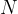$N$ coins,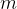$m$ of which are fair and the rest are biased. The probability of getting Head when a biased coin is tossed is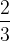$\dfrac{2}{3}$. A coin is drawn at random from the box and tossed twice. The first time it shows Head and the second time it shows Tail. What is the probability that the coin drawn is fair?
 Solution: 15
The probability tree for this problem is as follows:The required probability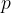$p$ is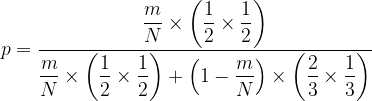$p = \dfrac{{\dfrac{m}{N} \times \left( {\dfrac{1}{2} \times \dfrac{1}{2}} \right)}}{{\dfrac{m}{N} \times \left( {\dfrac{1}{2} \times \dfrac{1}{2}} \right) + \left( {1 - \dfrac{m}{N}} \right) \times \left( {\dfrac{2}{3} \times \dfrac{1}{3}} \right)}}$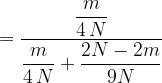$= \dfrac{{\dfrac{m}{{4\,N}}}}{{\dfrac{m}{{4\,N}} + \dfrac{{2N - 2m}}{{9N}}}}$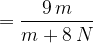$= \dfrac{{9\,m}}{{m + 8\,N}}$
In the terminology of Baye’s theorem, the expression for$p$ will be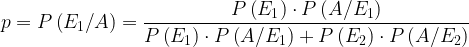$p = P\left( {{E_1}/A} \right) = \dfrac{{P\left( {{E_1}} \right) \cdot P\left( {A/{E_1}} \right)}}{{P\left( {{E_1}} \right) \cdot P\left( {A/{E_1}} \right) + P\left( {{E_2}} \right) \cdot P\left( {A/{E_2}} \right)}}$
 Example: 16
 A card from a pack of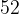$52$ cards is lost. From the remaining cards of the pack two cards are drawn at random and are found to be spades. Find the probability that the missing card was a spade.
 Solution: 16
Again, let us first approach the problem by drawing the corresponding probability tree:Observe carefully the probabilities of all the relevant steps.
The required probability$p$ is$p = \dfrac{{\dfrac{1}{4} \times \dfrac{{^{{\rm{12}}}{C_{\rm{2}}}}}{{^{{\rm{51}}}{C_{\rm{2}}}}}}}{{\dfrac{{\rm{1}}}{{\rm{4}}} \times \dfrac{{^{{\rm{12}}}{C_{\rm{2}}}}}{{^{{\rm{51}}}{C_{\rm{2}}}}} + \dfrac{3}{4} \times \dfrac{{^{{\rm{13}}}{C_{\rm{2}}}}}{{^{{\rm{51}}}{C_{\rm{2}}}}}}}$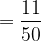$= \dfrac{{11}}{{50}}$
Hence, the chances of the missing card being a spade are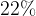$22\%$. Can you appreciate this answer? In particular, can you see why it is less than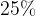$25\%$?